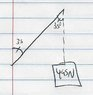# Resolution of Forces

brandon1
I can't figure out how to do this one:

"Mr. Porter has attached a sign that has a weight of 495N to a wall outside his home. Determine: a) The magnitude of the tension in the chain; b) The thrust force exerted by the rod, if the angle is 35 degrees"

I'm not seeing how the answers are a) 863N and b)706.9N (my teacher gave us the answers)

Homework Helper
Draw a diagram.
Weight is the y-component.

Mulder
Can you show us what you've done so far on getting the answers?

From the answers, the rod must be at 35 degrees to the wall, rather than the horizontal. It's really only two simple calculations, just draw a diagram of forces, all there is to it.

brandon1
What I'm not understanding is how the tension is 863N? If the signing is hanging straight down, shouldn't is be 495N [495sin(90)]?

Last edited:
Homework Helper
It isn't hanging straight down.

brandon1
Where do you see that?

Homework Helper
There's the sign, a chain, a rod and 35 degrees. I'd say the rod's placed horizontally.

brandon1
This is all I can get.

Physics is not exactly my best subject#### Attachments

•scan0001.jpg
13.9 KB · Views: 351
Last edited:
brandon1
That makes a lot more sense
Thanks